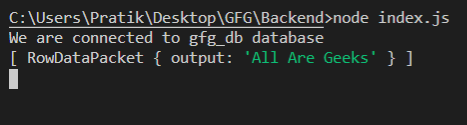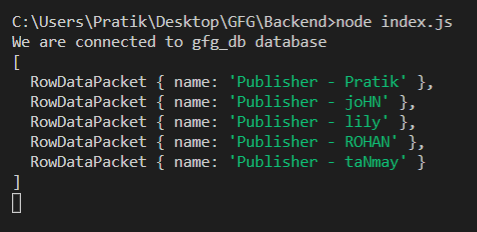Open In App

# Node.js MySQL INSERT() Function

INSERT() function is a built-in function in MySQL that is used to insert a string into another string at some position after deleting some characters from it.

Syntax:

`INSERT(string_1, position, number_of_characters_to_delete, string_2)`

Parameters: It takes four parameters as follows:

• string_1: It is the given main string passed as a parameter.
• position: The string_2 will insert at this position.
• number_of_characters_to_delete: It is the number of characters to be deleted from string_1 at a given position.
• string_2: It is the given new string for insertion.

Return Value: It returns a new string after deleting characters from string_1 then inserting string_2.

Module Installation: Install the mysql module using the following command:

`npm install mysql`

Database: Our SQL publishers table preview with sample data is shown below:Example 1:

## index.js

 `const mysql = require(``"mysql"``);`` ` `let db_con  = mysql.createConnection({``    ``host: ``"localhost"``,``    ``user: ``"root"``,``    ``password: ``''``,``    ``database: ``'gfg_db'``});`` ` `db_con.connect((err) => {``    ``if` `(err) {``      ``console.log(``"Database Connection Failed !!!"``, err);``      ``return``;``    ``}`` ` `    ``console.log(``"We are connected to gfg_db database"``);`` ` `    ``// Here is the query``    ``let query = ``"SELECT INSERT('GeeksforGeeks', 1, 8, 'All Are ') AS output"``;`` ` `    ``db_con.query(query, (err, rows) => {``        ``if``(err) ``throw` `err;`` ` `        ``console.log(rows);``    ``});``});`

Run the index.js file using the following command:

`node index.js`

Output: Here, the first 8 characters are deleted from ‘GeeksforGeeks’ from position 1 – ‘Geeks’. Then string_2 is inserted – ‘All Are Geeks’Example 2:

## index.js

 `const mysql = require(``"mysql"``);`` ` `let db_con  = mysql.createConnection({``    ``host: ``"localhost"``,``    ``user: ``"root"``,``    ``password: ``''``,``    ``database: ``'gfg_db'``});`` ` `db_con.connect((err) => {``    ``if` `(err) {``      ``console.log(``"Database Connection Failed !!!"``, err);``      ``return``;``    ``}`` ` `    ``console.log(``"We are connected to gfg_db database"``);`` ` `    ``// Here is the query``    ``let query = ``"SELECT INSERT(name, 1, 0, 'Publisher - ') AS name FROM publishers"``;`` ` `    ``db_con.query(query, (err, rows) => {``        ``if``(err) ``throw` `err;`` ` `        ``console.log(rows);``    ``});``});`

Run the index.js file using the following command:

`node index.js`

Output: